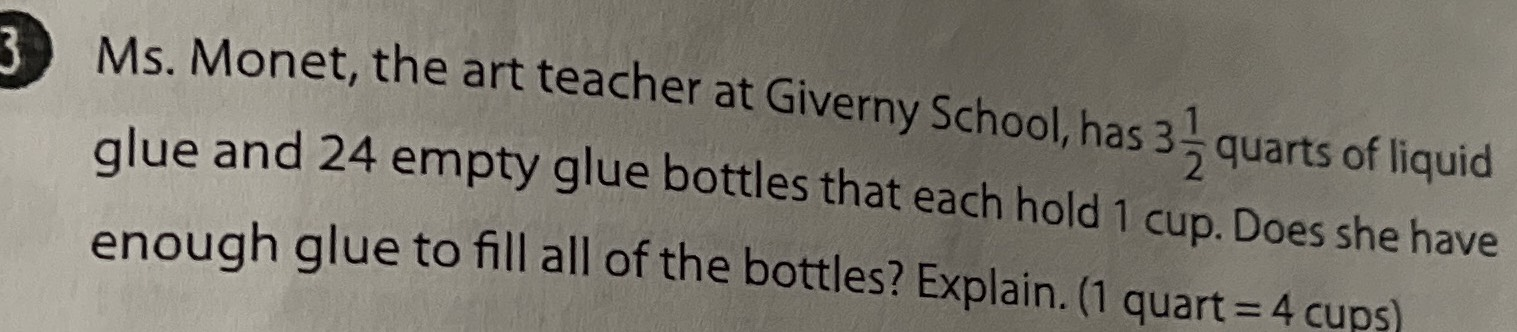### Still have math questions?

Arithmetic
Question3. Ms. Monet, the art teacher at Giverny School, has $$3 \frac { 1 } { 2 }$$ quarts of liquid glue and $$24$$ empty glue bottles that each hold $$1$$ cup. Does she have enough glue to fill all of the bottles? Explain. (1 quart $$= 4$$ cups)